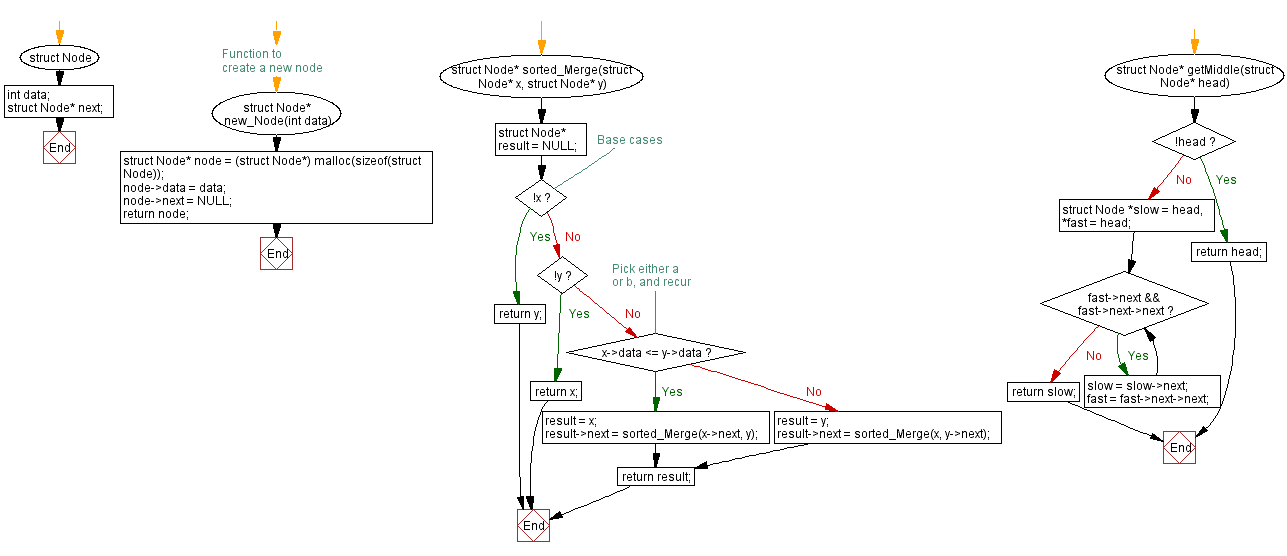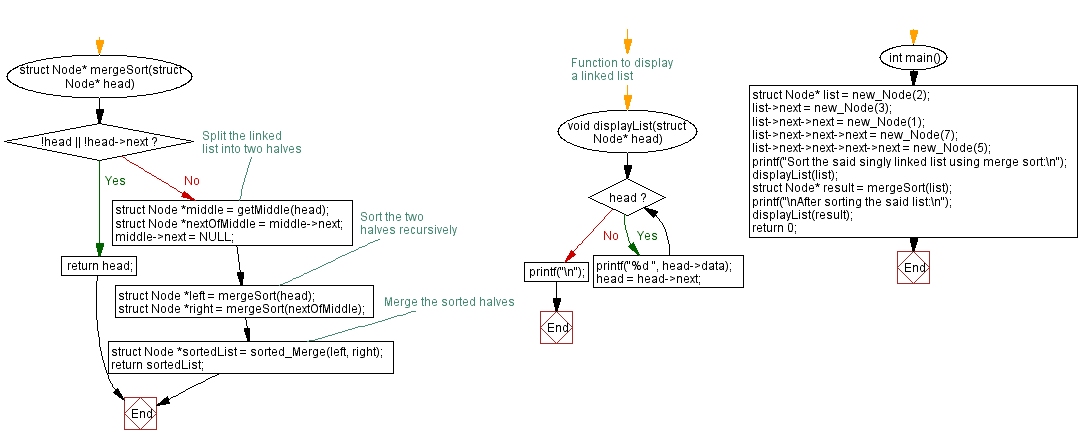﻿ C Program: Sort a singly linked list using merge sort

# C Exercises: Sort a singly linked list using merge sort

## C Singly Linked List : Exercise-17 with Solution

Write a C program to sort a singly linked list using merge sort.

Sample Solution:

C Code:

``````#include<stdio.h>
#include <stdlib.h>

struct Node {
int data;
struct Node* next;
};

// Function to create a new node
struct Node* new_Node(int data) {
struct Node* node = (struct Node*) malloc(sizeof(struct Node));
node->data = data;
node->next = NULL;
return node;
}

struct Node* sorted_Merge(struct Node* x, struct Node* y) {
struct Node* result = NULL;

// Base cases
if (!x)
return y;
if (!y)
return x;

// Pick either a or b, and recur
if (x->data <= y->data) {
result = x;
result->next = sorted_Merge(x->next, y);
} else {
result = y;
result->next = sorted_Merge(x, y->next);
}
return result;
}

struct Node* getMiddle(struct Node* head) {

while (fast->next && fast->next->next) {
slow = slow->next;
fast = fast->next->next;
}
return slow;
}

struct Node* mergeSort(struct Node* head) {

// Split the linked list into two halves
struct Node *nextOfMiddle = middle->next;
middle->next = NULL;

// Sort the two halves recursively
struct Node *right = mergeSort(nextOfMiddle);

// Merge the sorted halves
struct Node *sortedList = sorted_Merge(left, right);
return sortedList;
}

// Function to display a linked list
}
printf("\n");
}

int main() {
struct Node* list = new_Node(2);
list->next = new_Node(3);
list->next->next = new_Node(1);
list->next->next->next = new_Node(7);
list->next->next->next->next = new_Node(5);
printf("Sort the said singly linked list using merge sort:\n");
displayList(list);
struct Node* result = mergeSort(list);
printf("\nAfter sorting the said list:\n");
displayList(result);
return 0;
}
```
```

Sample Output:

```Sort the said singly linked list using merge sort:
2 3 1 7 5

After sorting the said list:
1 2 3 5 7
```

Flowchart :C Programming Code Editor:

What is the difficulty level of this exercise?

﻿